# Chapter 4 Chang ChiChung 2007 5 17 The

• Slides: 59
Download presentation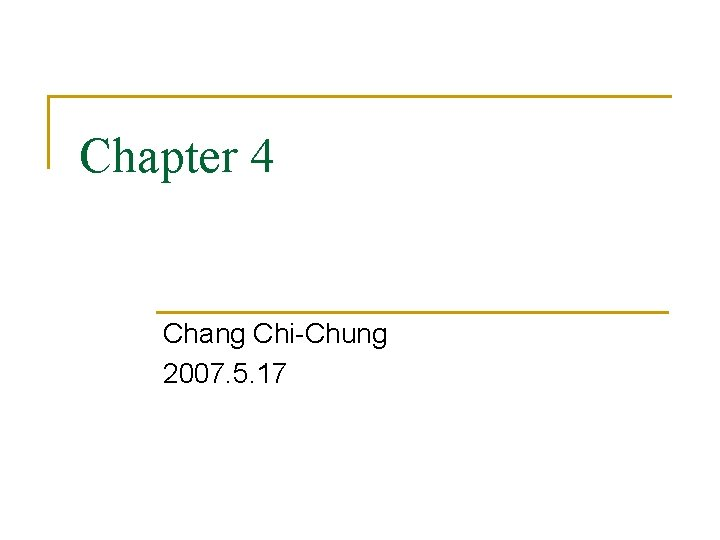Chapter 4 Chang Chi-Chung 2007. 5. 17The Role of the Parser Source Program Lexical Analyzer Token Parser get. Next. Token Parse tree Symbol Table Rest of Front intermediate representation End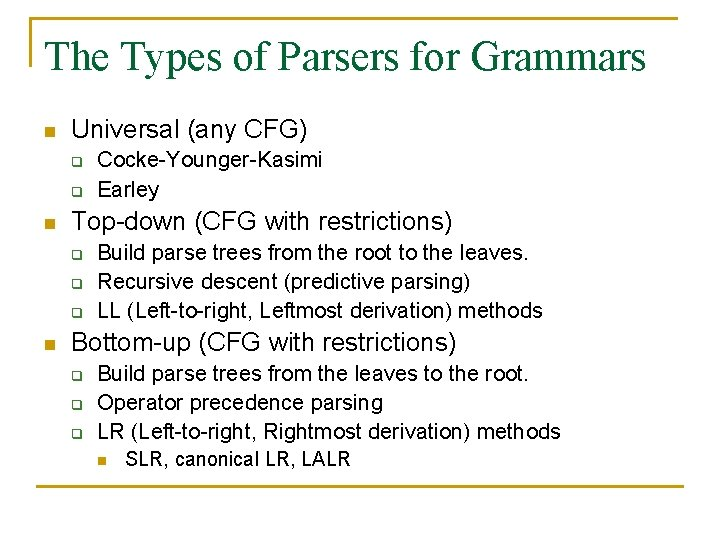The Types of Parsers for Grammars n Universal (any CFG) q q n Top-down (CFG with restrictions) q q q n Cocke-Younger-Kasimi Earley Build parse trees from the root to the leaves. Recursive descent (predictive parsing) LL (Left-to-right, Leftmost derivation) methods Bottom-up (CFG with restrictions) q q q Build parse trees from the leaves to the root. Operator precedence parsing LR (Left-to-right, Rightmost derivation) methods n SLR, canonical LR, LALR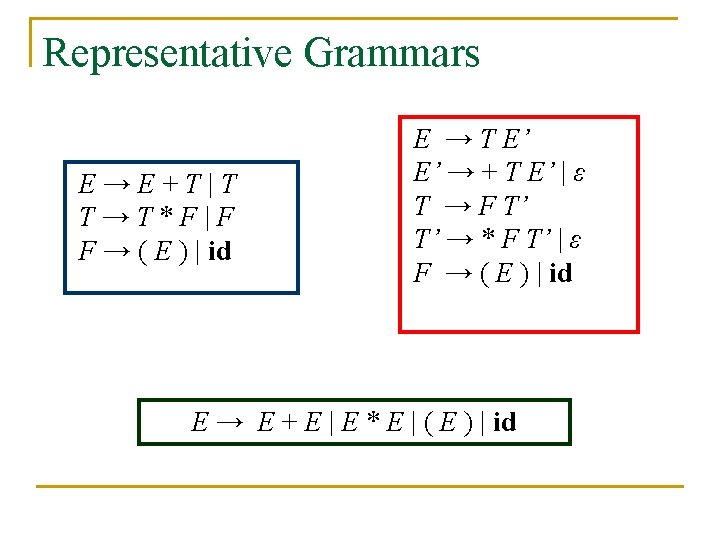Representative Grammars E→E+T|T T→T*F|F F → ( E ) | id E → T E’ E’ → + T E’ | ε T → F T’ T’ → * F T’ | ε F → ( E ) | id E → E + E | E * E | ( E ) | idError Handling n A good compiler should assist in identifying and locating errors q Lexical errors n q Syntax errors n q important, can sometimes recover Dynamic semantic errors n q most important for compiler, can almost always recover Static semantic errors n q important, compiler can easily recover and continue hard or impossible to detect at compile time, runtime checks are required Logical errors n hard or impossible to detectError Recovery Strategies n Panic mode q n Phrase-level recovery q n Perform local correction on the input to repair the error Error productions q n Discard input until a token in a set of designated synchronizing tokens is found Augment grammar with productions for erroneous constructs Global correction q Choose a minimal sequence of changes to obtain a global least-cost correctionContext-Free Grammar n Context-free grammar is a 4 -tuple G = < T, N, P, S> where q q T is a finite set of tokens (terminal symbols) N is a finite set of nonterminals P is a finite set of productions of the form where N and (N T)* S N is a designated start symbol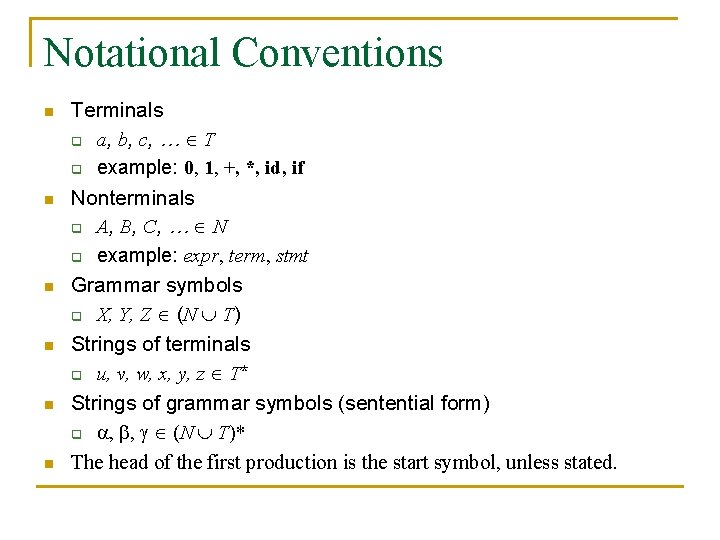Notational Conventions n n n Terminals q a, b, c, … T q example: 0, 1, +, *, id, if Nonterminals q A, B, C, … N q example: expr, term, stmt Grammar symbols q X, Y, Z (N T) Strings of terminals q u, v, w, x, y, z T* Strings of grammar symbols (sentential form) q , , (N T)* The head of the first production is the start symbol, unless stated.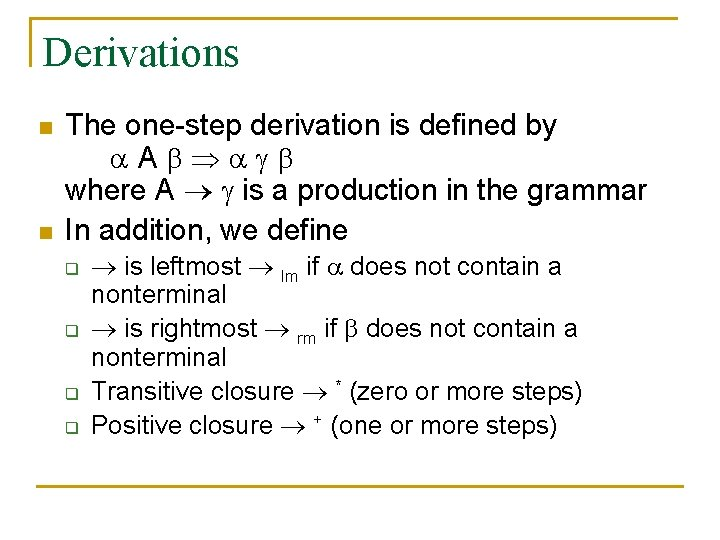Derivations n n The one-step derivation is defined by A where A is a production in the grammar In addition, we define q q is leftmost lm if does not contain a nonterminal is rightmost rm if does not contain a nonterminal Transitive closure * (zero or more steps) Positive closure + (one or more steps)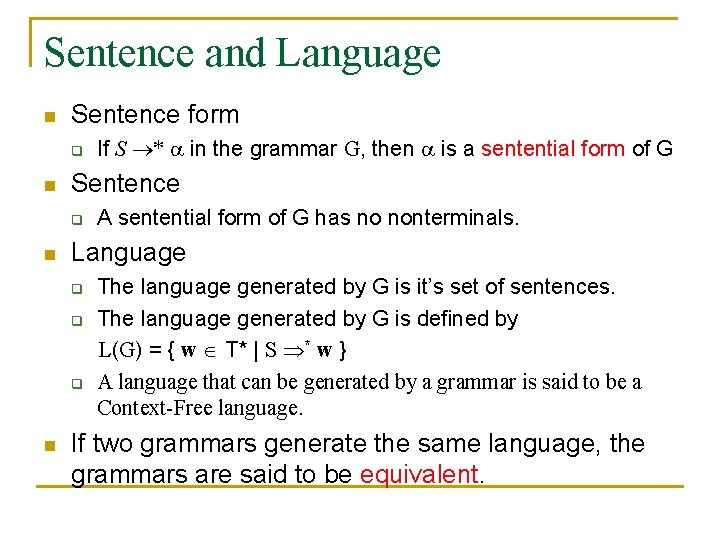Sentence and Language n Sentence form q n Sentence q n A sentential form of G has no nonterminals. Language q q q n If S * in the grammar G, then is a sentential form of G The language generated by G is it’s set of sentences. The language generated by G is defined by L(G) = { w T* | S * w } A language that can be generated by a grammar is said to be a Context-Free language. If two grammars generate the same language, the grammars are said to be equivalent.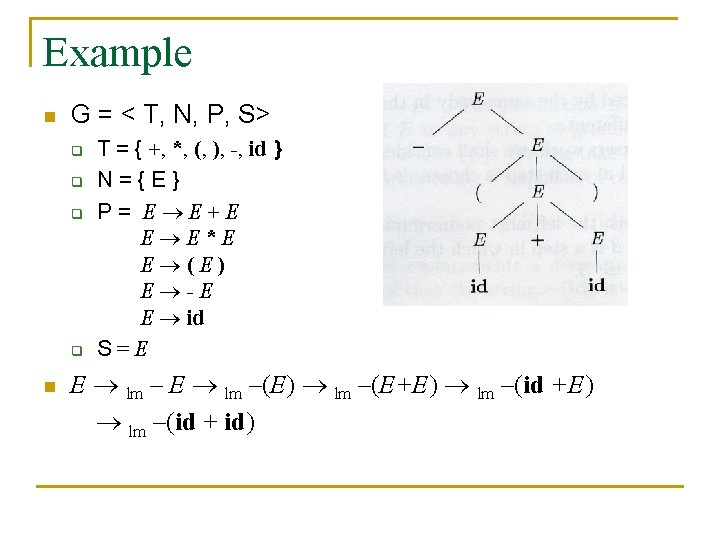Example n G = < T, N, P, S> q q n T = { +, *, (, ), -, id } N={E} P= E E+E E E*E E (E) E -E E id S=E E lm –(E) lm –(E+E) lm –(id + id)Ambiguity n n A grammar that produces more than one parse tree for some sentence is said to be ambiguous. Example q id + id * id E → E + E | E * E | ( E ) | id E E+E id + E * E id + id * id E E*E E+E*E id + E * E id + id * id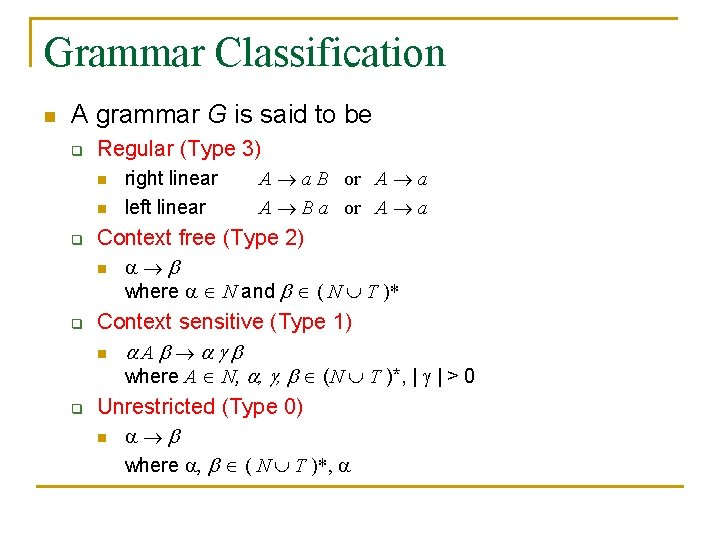Grammar Classification n A grammar G is said to be q Regular (Type 3) n n q q q right linear left linear A a B or A a A B a or A a Context free (Type 2) n where N and ( N T )* Context sensitive (Type 1) n A where A N, , , (N T )*, | | > 0 Unrestricted (Type 0) n where , ( N T )*,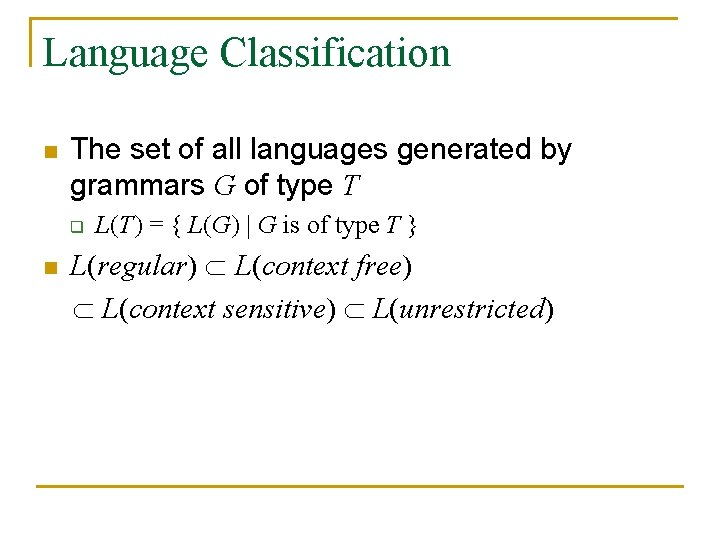Language Classification n The set of all languages generated by grammars G of type T q n L(T) = { L(G) | G is of type T } L(regular) L(context free) L(context sensitive) L(unrestricted)Example ( a | b )* a b b a A 0 a A 1 b A 2 b A 0 a. A 0 | b. A 0 | a. A 1 b. A 2 b. A 3 ε b A 3Example n L = { anbn | n 1 } is context free. path labeled aj-i S 0 … path labeled ai Si … f path labeled bi ajbi can be accepted by D, but ajbi is not in the L. Finite automata cannot count. CFG can count two items but not three.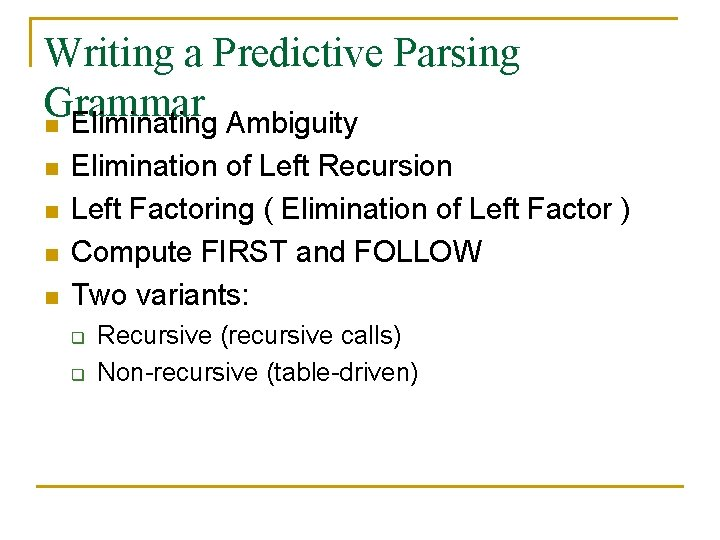Writing a Predictive Parsing Grammar n Eliminating Ambiguity n n Elimination of Left Recursion Left Factoring ( Elimination of Left Factor ) Compute FIRST and FOLLOW Two variants: q q Recursive (recursive calls) Non-recursive (table-driven)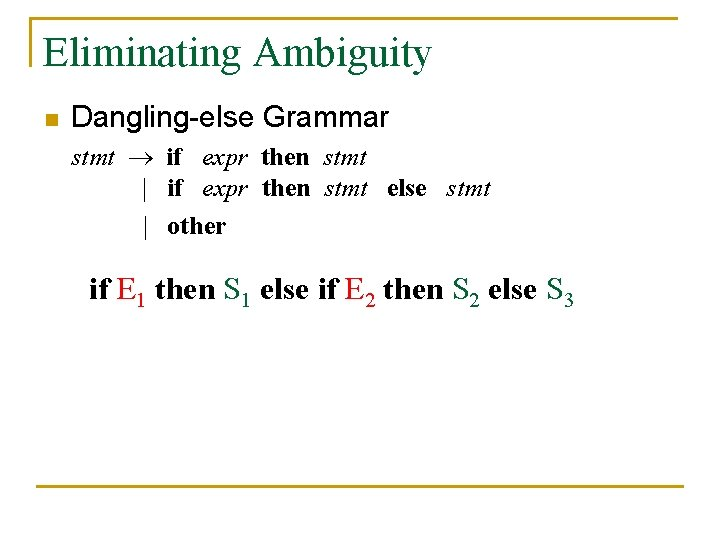Eliminating Ambiguity n Dangling-else Grammar stmt if expr then stmt | if expr then stmt else stmt | other if E 1 then S 1 else if E 2 then S 2 else S 3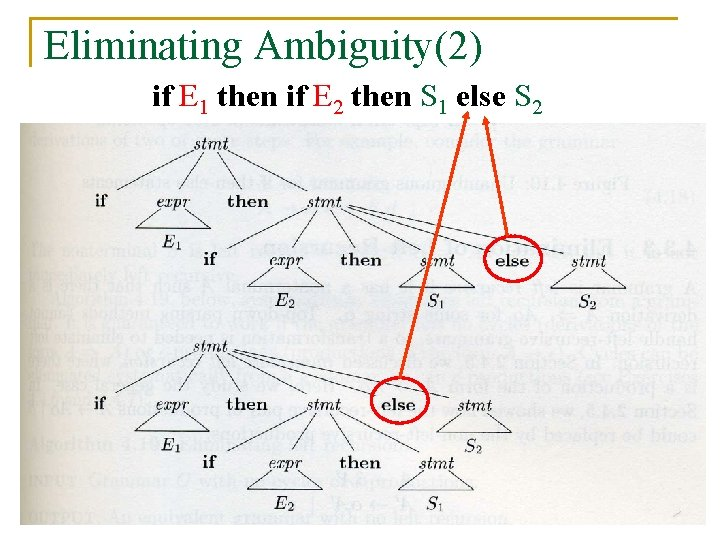Eliminating Ambiguity(2) if E 1 then if E 2 then S 1 else S 2Eliminating Ambiguity(3) n Rewrite the dangling-else grammar stmt matched_stmt | open_stmt matched_stmt if expr then matched_stmt else matched_stmt | other open_stmt if expr then stmt | if expr then matched_stmt else open_stmtElimination of Left Recursion n n Productions of the form A A | are left recursive Non-left-recursions A A’ A’ A’ | ε n When one of the productions in a grammar is left recursive then a predictive parser loops forever on certain inputs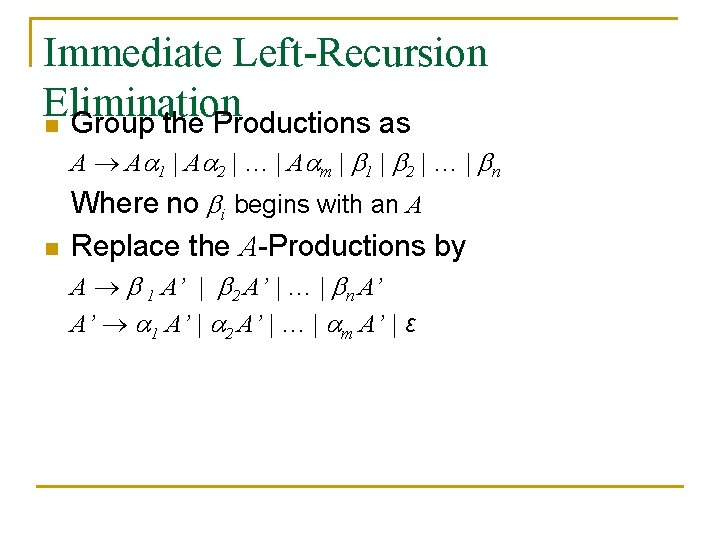Immediate Left-Recursion Elimination n Group the Productions as A A 1 | A 2 | … | A m | 1 | 2 | … | n n Where no i begins with an A Replace the A-Productions by A 1 A’ | 2 A’ | … | n A’ A’ 1 A’ | 2 A’ | … | m A’ | ε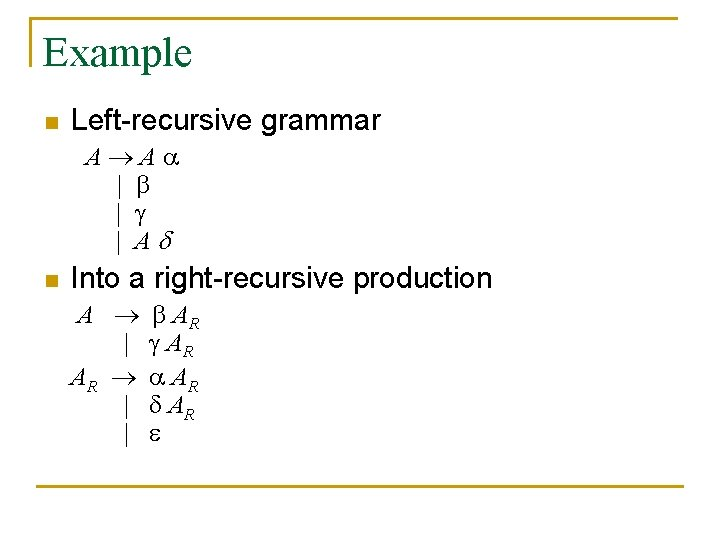Example n Left-recursive grammar A A | | | A n Into a right-recursive production A | AR | | AR AR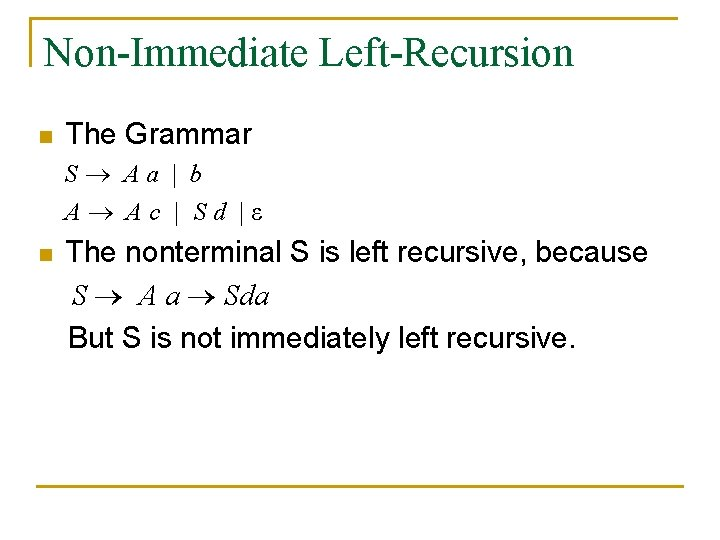Non-Immediate Left-Recursion n The Grammar S Aa | b A Ac | Sd |ε n The nonterminal S is left recursive, because S A a Sda But S is not immediately left recursive.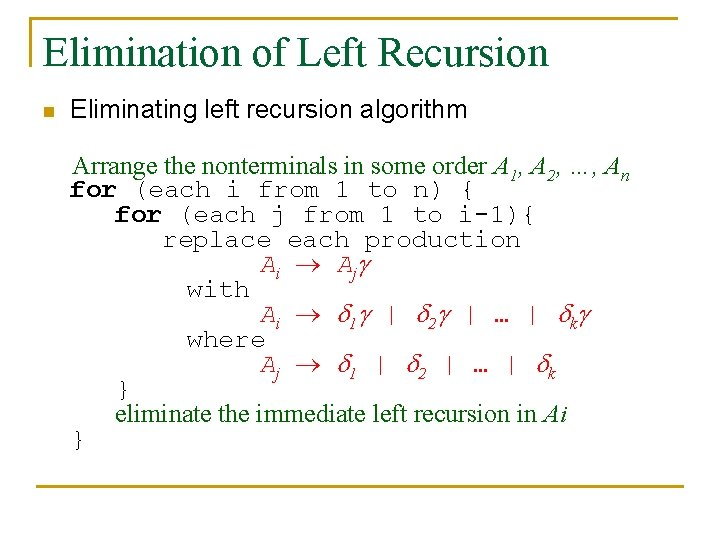Elimination of Left Recursion n Eliminating left recursion algorithm Arrange the nonterminals in some order A 1, A 2, …, An for (each i from 1 to n) { for (each j from 1 to i-1){ replace each production Ai Aj with Ai 1 | 2 | … | k where Aj 1 | 2 | … | k } eliminate the immediate left recursion in Ai }Example A BC|a B CA|Ab C AB|CC|a i=1 nothing to do i = 2, j = 1 B CA|Ab B CA|BCb|ab (imm) B C A BR | a b BR BR C b BR | i = 3, j = 1 C AB|CC|a C BCB|a. B|CC|a i = 3, j = 2 C BCB|a. B|CC|a C C A BR C B | a b BR C B | a B | C C | a (imm)C a b BR C B CR | a CR CR A B R C B C R | C C R |Exercise n The grammar S Aa | b A Ac | Sd |ε n Answer q A Ac|Aad|bd|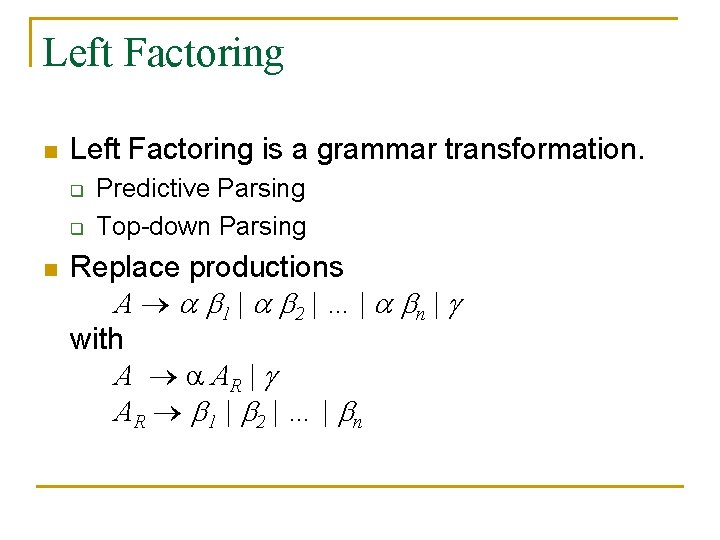Left Factoring n Left Factoring is a grammar transformation. q q n Predictive Parsing Top-down Parsing Replace productions A 1 | 2 | … | n | with A AR | AR 1 | 2 | … | n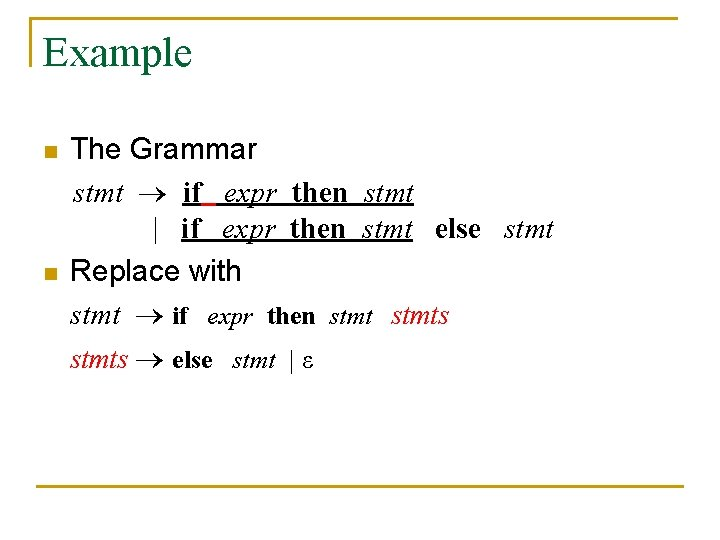Example n n The Grammar stmt if expr then stmt | if expr then stmt else stmt Replace with stmt if expr then stmts else stmt | ε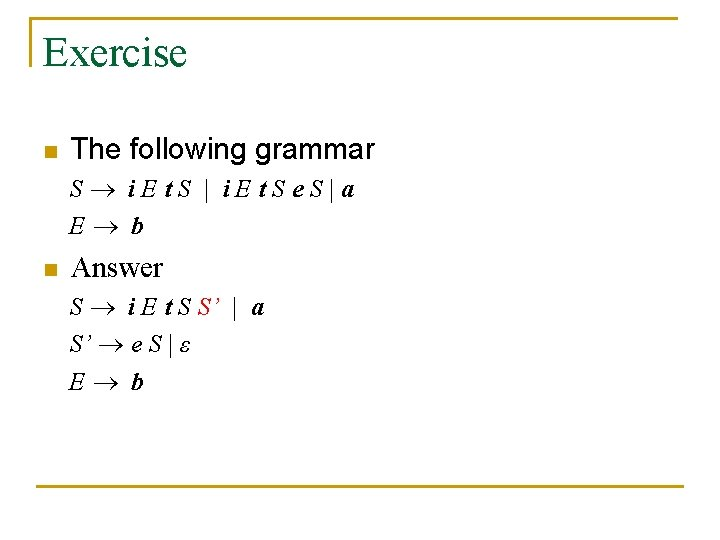Exercise n The following grammar S i. Et. S | i. Et. Se. S|a E b n Answer S i E t S S’ | a S’ e S | ε E bNon-Context-Free Grammar Constructs n n A few syntactic constructs found in typical programming languages cannot be specified using grammars alone. Checking the identifiers are declared before they are used in a program. q q The abstract language is L 1 = { wcw | w is in (a|b)* } aabcaab is in L 1 and L 1 is not CFG. C/C++ and Java does not distinguish among identifiers that are different character strings. All identifiers are represented by a token such as id in a grammar. In the semantic-analysis phase checks that identifiers are declared before they are used.Top-Down Parsing n LL methods and recursive-descent parsing q q Left-to-right, Leftmost derivation Creating the nodes of the parse tree in preorder ( depthfirst ) Grammar E T+T T (E) T -E T id E Leftmost derivation E lm T + T lm id + id E E T T + T id + E T T T id + id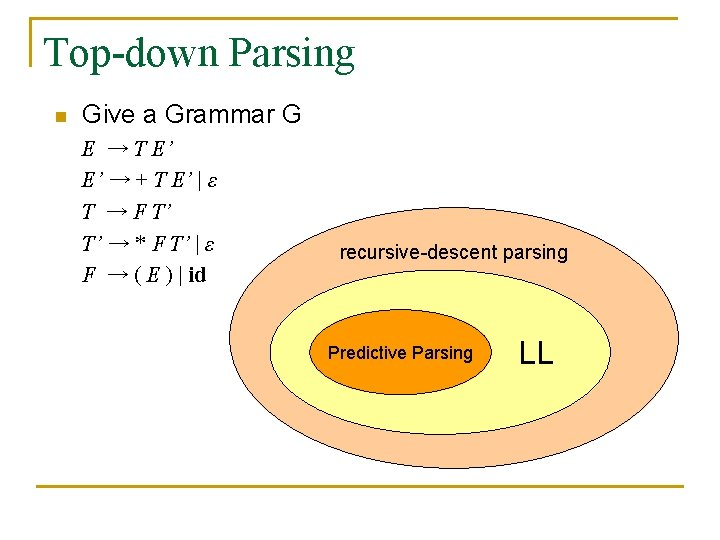Top-down Parsing n Give a Grammar G E → T E’ E’ → + T E’ | ε T → F T’ T’ → * F T’ | ε F → ( E ) | id recursive-descent parsing Predictive Parsing LL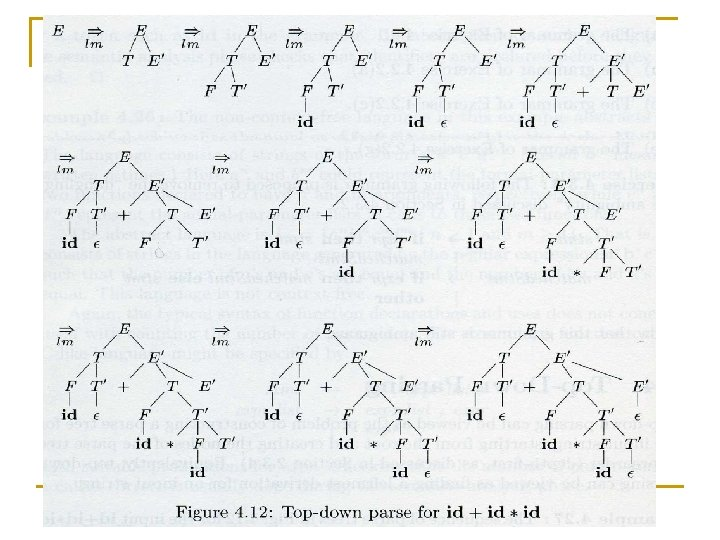FIRST and FOLLOW S a A α c β γ c is in FIRST(A) a is in FOLLOW(A)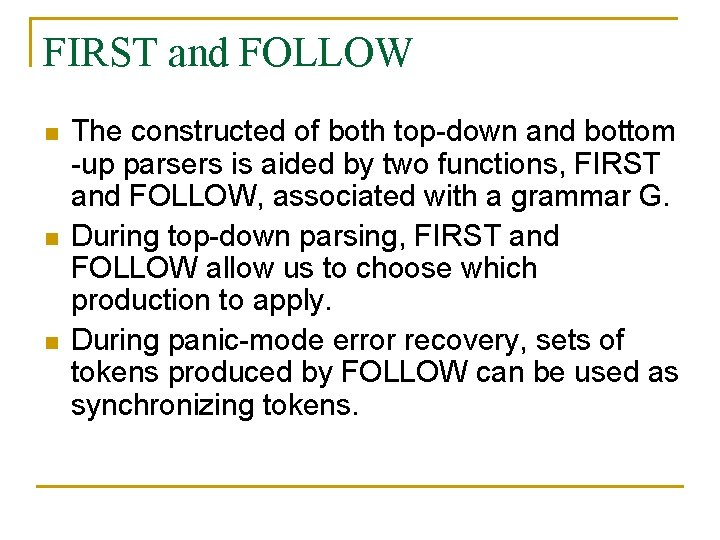FIRST and FOLLOW n n n The constructed of both top-down and bottom -up parsers is aided by two functions, FIRST and FOLLOW, associated with a grammar G. During top-down parsing, FIRST and FOLLOW allow us to choose which production to apply. During panic-mode error recovery, sets of tokens produced by FOLLOW can be used as synchronizing tokens.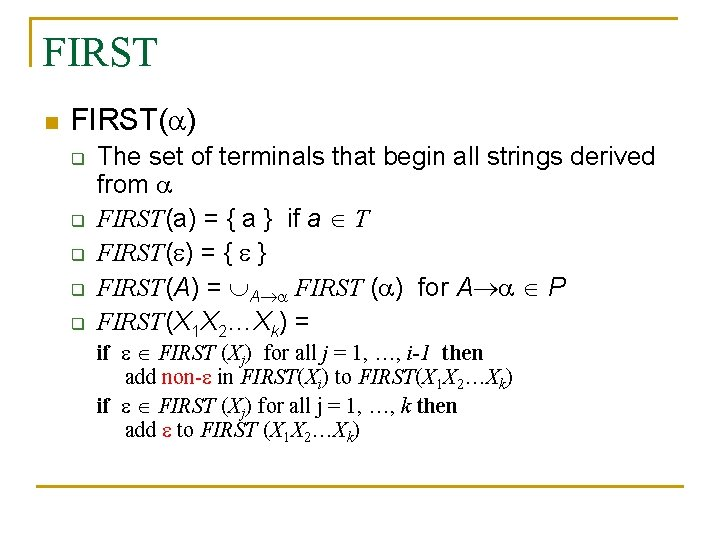FIRST n FIRST( ) q q q The set of terminals that begin all strings derived from FIRST(a) = { a } if a T FIRST( ) = { } FIRST(A) = A FIRST ( ) for A P FIRST(X 1 X 2…Xk) = if FIRST (Xj) for all j = 1, …, i-1 then add non- in FIRST(Xi) to FIRST(X 1 X 2…Xk) if FIRST (Xj) for all j = 1, …, k then add to FIRST (X 1 X 2…Xk)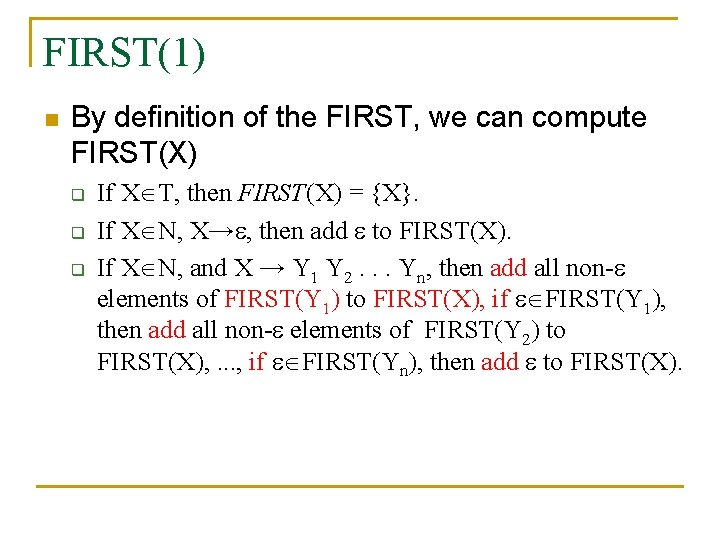FIRST(1) n By definition of the FIRST, we can compute FIRST(X) q q q If X T, then FIRST(X) = {X}. If X N, X→ , then add to FIRST(X). If X N, and X → Y 1 Y 2. . . Yn, then add all non- elements of FIRST(Y 1) to FIRST(X), if FIRST(Y 1), then add all non- elements of FIRST(Y 2) to FIRST(X), . . . , if FIRST(Yn), then add to FIRST(X).FOLLOW n FOLLOW(A) q q the set of terminals that can immediately follow nonterminal A FOLLOW(A) = for all (B A ) P do add FIRST( )-{ } to FOLLOW(A) for all (B A ) P and FIRST( ) do add FOLLOW(B) to FOLLOW(A) for all (B A) P do add FOLLOW(B) to FOLLOW(A) if A is the start symbol S then add \$ to FOLLOW(A)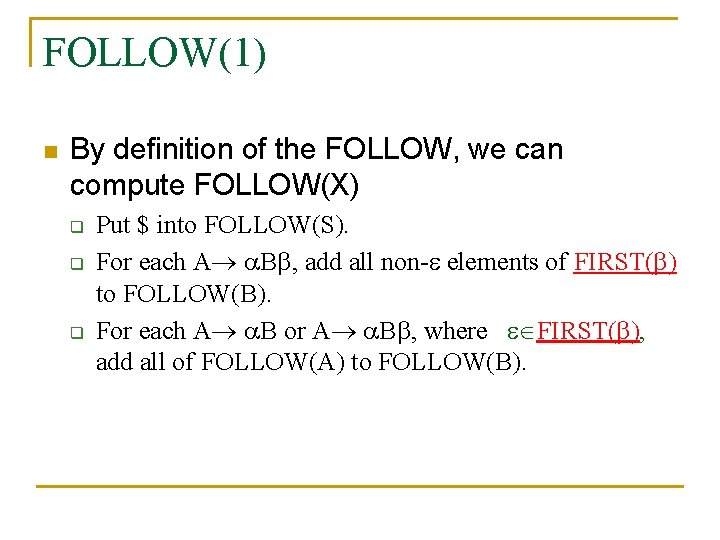FOLLOW(1) n By definition of the FOLLOW, we can compute FOLLOW(X) q q q Put \$ into FOLLOW(S). For each A B , add all non- elements of FIRST( ) to FOLLOW(B). For each A B or A B , where FIRST( ), add all of FOLLOW(A) to FOLLOW(B).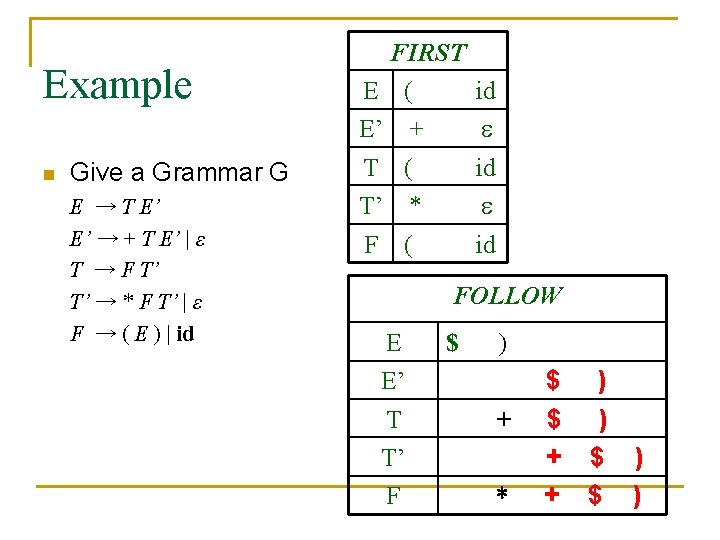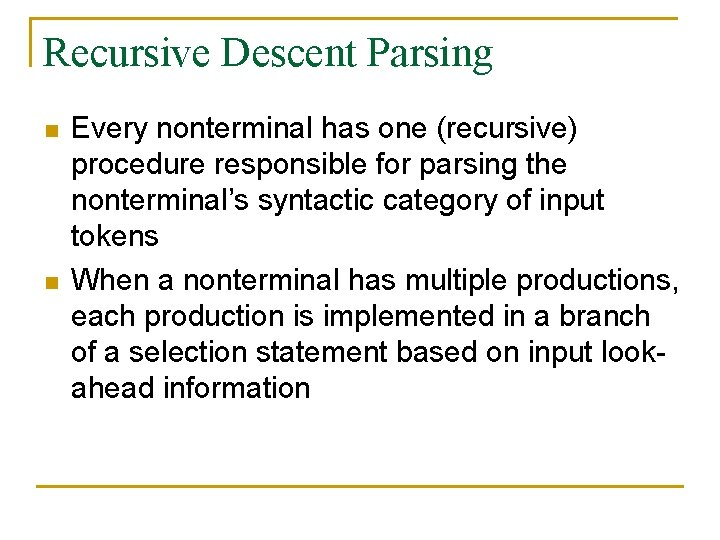Recursive Descent Parsing n n Every nonterminal has one (recursive) procedure responsible for parsing the nonterminal’s syntactic category of input tokens When a nonterminal has multiple productions, each production is implemented in a branch of a selection statement based on input lookahead information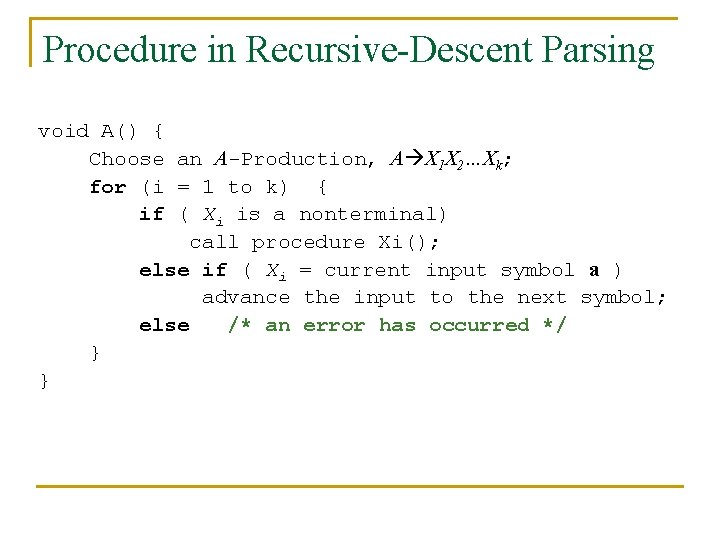Procedure in Recursive-Descent Parsing void A() { Choose an A-Production, A X 1 X 2…Xk; for (i = 1 to k) { if ( Xi is a nonterminal) call procedure Xi(); else if ( Xi = current input symbol a ) advance the input to the next symbol; else /* an error has occurred */ } }Using FIRST and FOLLOW to Write a Recursive Descent Parser expr term rest + term rest | - term rest | term id rest() { if (lookahead in FIRST(+ term rest) ) { match(‘+’); term(); rest() } else if (lookahead in FIRST(- term rest) ) { match(‘-’); term(); rest() } else if (lookahead in FOLLOW(rest) ) return else error() } FIRST(+ term rest) = { + } FIRST(- term rest) = { - } FOLLOW(rest) = { \$ }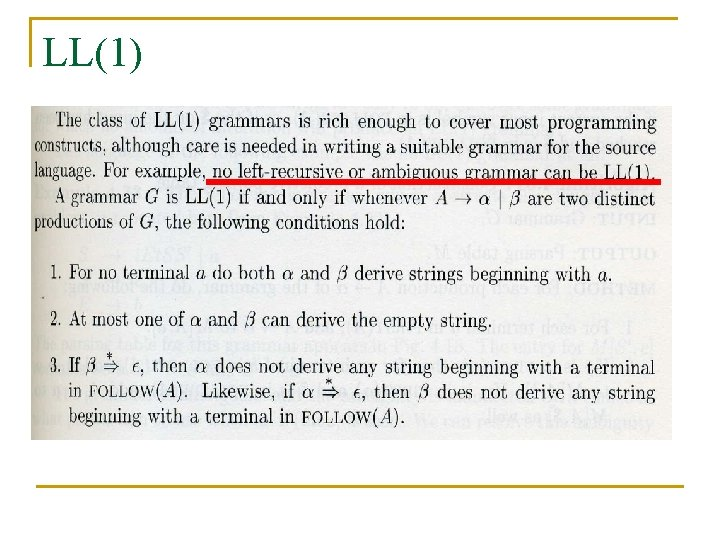LL(1)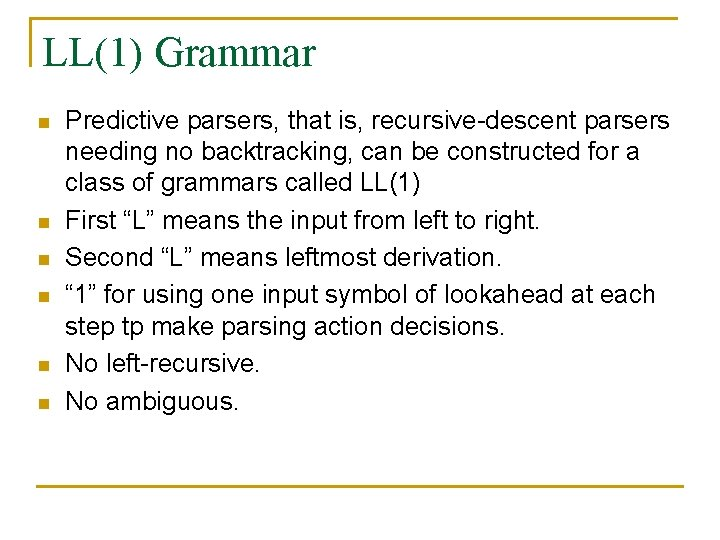LL(1) Grammar n n n Predictive parsers, that is, recursive-descent parsers needing no backtracking, can be constructed for a class of grammars called LL(1) First “L” means the input from left to right. Second “L” means leftmost derivation. “ 1” for using one input symbol of lookahead at each step tp make parsing action decisions. No left-recursive. No ambiguous.LL(1) n A grammar G is LL(1) if it is not left recursive and for each collection of productions A 1 | 2 | … | n for nonterminal A the following holds: q q FIRST( i) FIRST( j) = for all i j 如果交集不是空集合，會如何？ if i * then n n j * for all i j FIRST( j) FOLLOW(A) = for all i jExample Grammar Not LL(1) because: S Sa|a Left recursive S a. S|a FIRST(a S) FIRST(a) ={a} S a. R| R S| For R: S * and * S a. Ra R S| For R: FIRST(S) FOLLOW(R)Non-Recursive Predictive Parsing n n Table-Driven Parsing Given an LL(1) grammar G = construct a table M[A, a] for A N, a T and use a driver program with a stack input a + b \$ stack X Y Z \$ Predictive parsing program (driver) Parsing table M outputPredictive Parsing Table Algorithm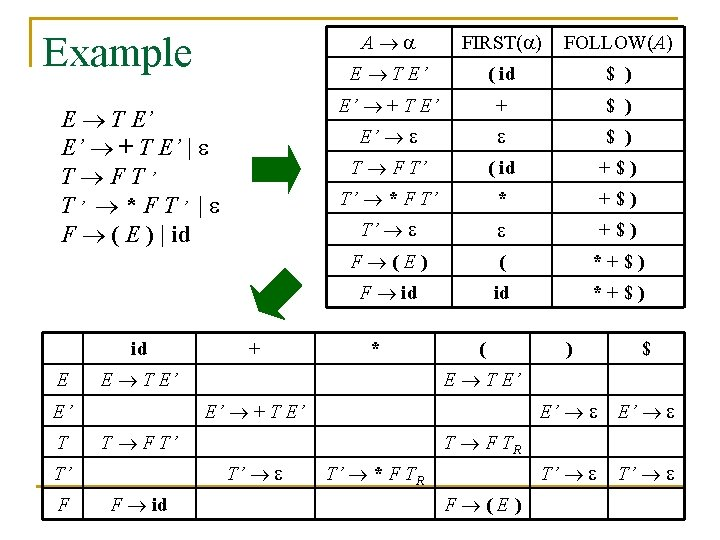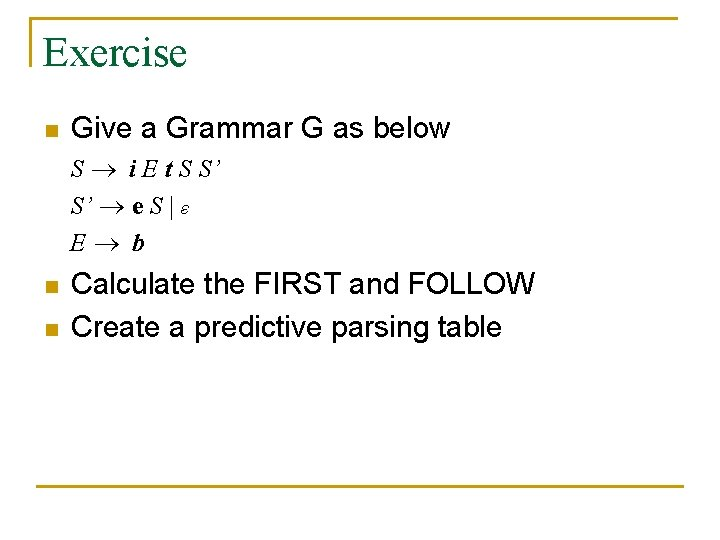Exercise n Give a Grammar G as below S i E t S S’ S’ e S | ε E b n n Calculate the FIRST and FOLLOW Create a predictive parsing table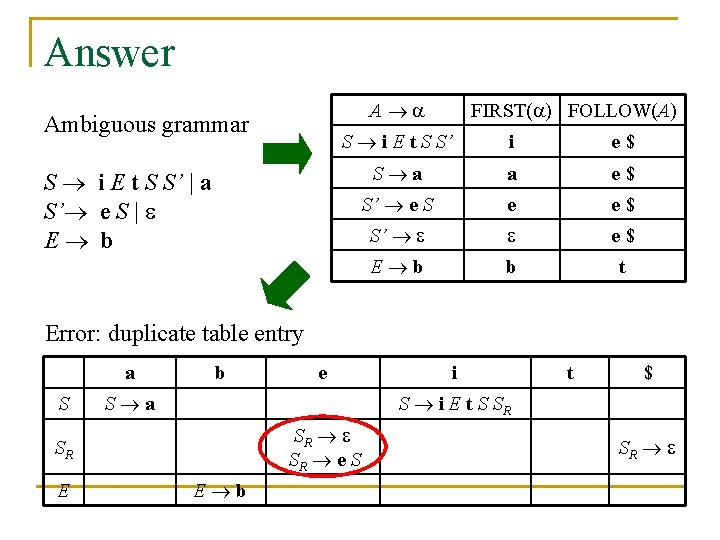Answer A Ambiguous grammar FIRST( ) FOLLOW(A) S i E t S S’ i e\$ S a a e\$ S’ e S e e\$ S’ e\$ E b b t S i E t S S’ | a S’ e S | E b Error: duplicate table entry a S b S a i t \$ S i E t S SR SR SR e S SR E e E b SRPredictive Parsing Algorithm Initially, w\$ is in the input buffer and S is on top of the stack set ip to point to the first symbol of w; set X to the top stack symbol; while (X != \$) { if (X is a) pop the stack and advance ip; else if ( X is a terminal) error(); else if ( M[X, a] is an error entry) error(); else if ( M[X, a] = X Y 1 Y 2…Yk ) { output the production X Y 1 Y 2…Yk pop the stack; push Yk , Yk-1 , …. Y 1 onto the stack, with Y 1 on top; } set X to the top stack symbol; }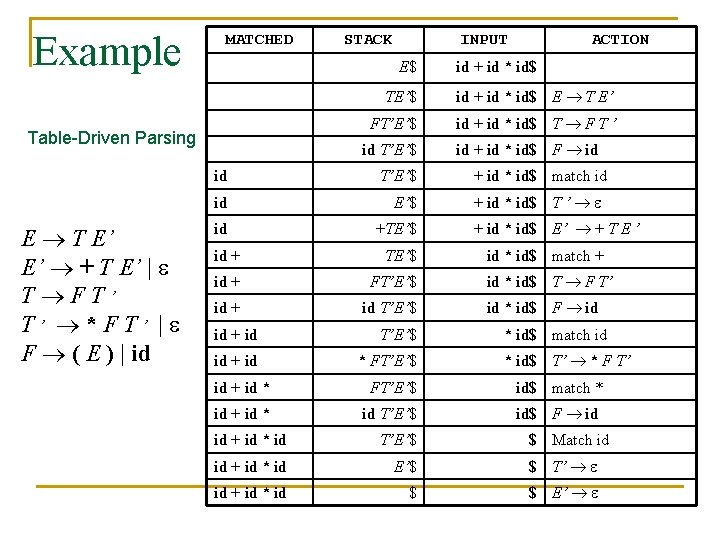Example MATCHED INPUT E\$ Table-Driven Parsing E T E’ E’ + T E’ | T FT’ T’ * F T’ | F ( E ) | id STACK ACTION id + id * id\$ TE’\$ id + id * id\$ E T E’ FT’E’\$ id + id * id\$ T F T ’ id T’E’\$ id +TE’\$ id + FT’E’\$ id + id * FT’E’\$ id + id * id\$ F id + id * id\$ match id + id * id\$ T ’ + id * id\$ E’ + T E ’ id * id\$ match + id * id\$ T F T’ id * id\$ F id * id\$ match id * id\$ T’ * F T’ id + id * FT’E’\$ id\$ match * id + id * id T’E’\$ id\$ F id id + id * id T’E’\$ \$ Match id id + id * id E’\$ \$ T’ id + id * id \$ \$ E’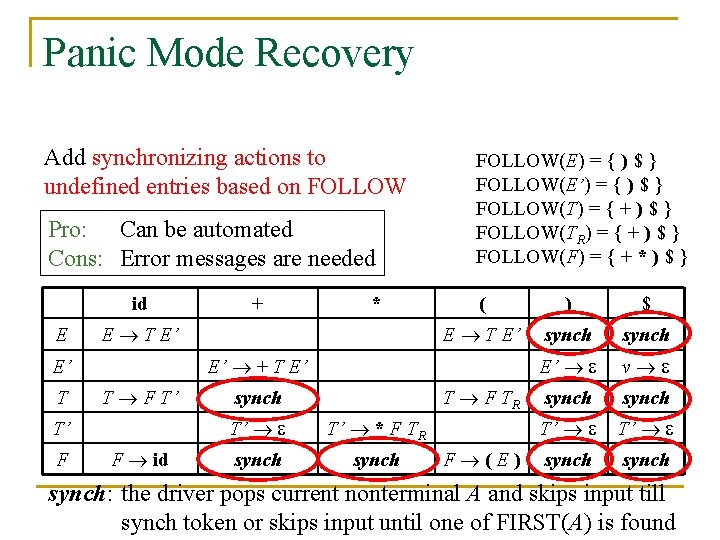Panic Mode Recovery Add synchronizing actions to undefined entries based on FOLLOW Pro: Can be automated Cons: Error messages are needed id E ( ) \$ E T E’ synch E’ v synch T’ T’ synch E’ + T E’ T F T’ T’ F * E T E’ E’ T + FOLLOW(E) = { ) \$ } FOLLOW(E’) = { ) \$ } FOLLOW(T) = { + ) \$ } FOLLOW(TR) = { + ) \$ } FOLLOW(F) = { + * ) \$ } F id T F TR synch T’ T’ * F TR synch F (E) synch: the driver pops current nonterminal A and skips input till synch token or skips input until one of FIRST(A) is foundExample STACK INPUT E\$ E\$ E T E’ E’ + T E’ | T FT’ T’ * F T’ | F ( E ) | id ACTION ) id * + id \$ error, skip ) id * + id \$ id is in FIRST(E) TE’\$ id * + id \$ FT’E’\$ id * + id \$ id T’E’\$ id * + id \$ T’E’\$ * + id \$ * FT’E’\$ * + id \$ FT’E’\$ + id \$ error, M[F, +] = synch + id \$ F has been popped E’\$ + id \$ +TE’\$ + id \$ T’E’\$ id \$ FT’E’\$ id \$ T’E’\$ \$ \$ \$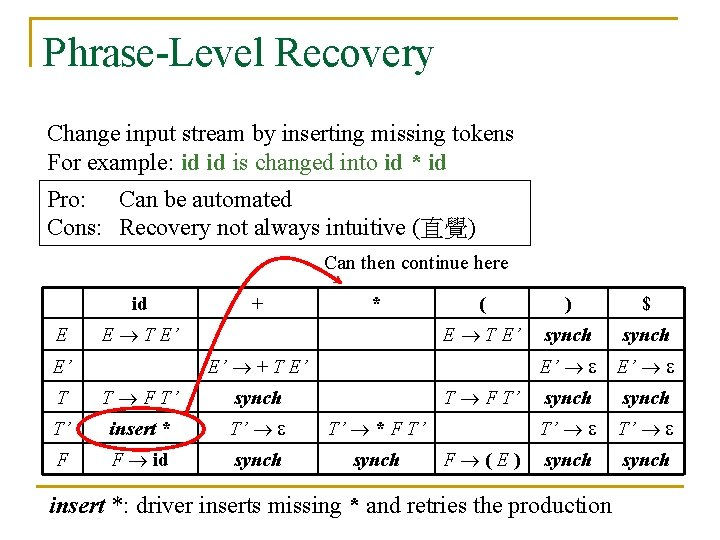Phrase-Level Recovery Change input stream by inserting missing tokens For example: id id is changed into id * id Pro: Can be automated Cons: Recovery not always intuitive (直覺) Can then continue here id E + * E T E’ ( ) \$ E T E’ synch E’ E’ synch T’ T’ synch E’ + T E’ E’ T T F T’ synch T F T’ T’ insert * T’ T’ * F T’ F F id synch F (E) insert *: driver inserts missing * and retries the productionError Productions Add “error production”: T’ F T ’ to ignore missing *, e. g. : id id E T E’ E’ + T E’ | T F T’ T’ * F T’ | F ( E ) | id id E Pro: Powerful recovery method Cons: Cannot be automated + * E T E’ ( ) \$ E T E’ synch E’ E’ synch T’ T’ synch E’ + T E’ E’ T T F T’ synch T F T’ T’ T’ * F TR F F id synch F (E)